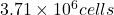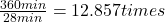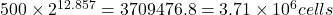## Proteus vulgaris has a doubling time of roughly 28 minutes. If an initial population of 500 cells is allowed to grow for 6 hours in ideal co

Question

Proteus vulgaris has a doubling time of roughly 28 minutes. If an initial population of 500 cells is allowed to grow for 6 hours in ideal conditions, what will be the final population? Use three significant figures for your final answer.

in progress 0
6 months 2021-07-23T09:10:52+00:00 1 Answers 18 views 0

## Answers ( )

1. Answer: The final population of Proteus vulgaris after 6 hours isExplanation:

We are given:

Proteus vulgaris divides and doubles every 28 minutes

Total time given = 6 hours = 360 min      (Conversion factor: 1 hr = 60 min)

Number of times Proteus vulgaris doubles in 6 hours =Calculating the number of bacteria after 6 hours under ideal conditions:

We are given:

Initial population = 500 cells

Number of times it doubles = 12.857 times

Final Proteus vulgaris population =Hence, the final population of Proteus vulgaris after 6 hours is